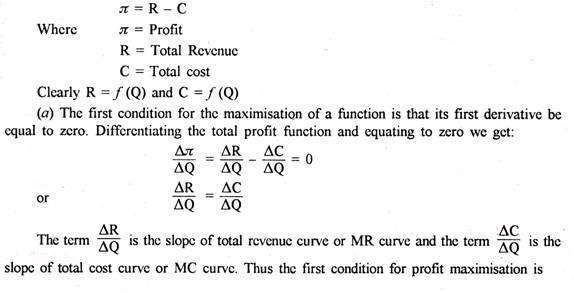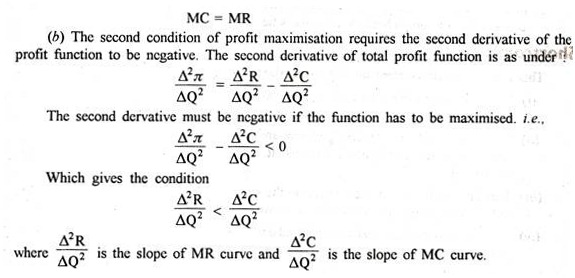Profit Maximisation Hypothesis of Traditional Economic Theory!

According to traditional economic theory profit maximisation is the sole objective of business firms. Profit maximisation means the largest absolute amount of money profits in given demand and supply conditions.

Conventional price theory is based upon profit maximisation hypothesis.

Profit maximisation hypothesis helps not only in predicting the behaviour of business firms but also the price-output behaviour under different market conditions.

No other hypothesis can explain and forecast the behaviours of firms better than this hypothesis. Under perfect competition individual firms have to maximise their profits at price determined by industry. Under imperfect competition firms search their profit maximising price output as they are price makers. The profit can be defined as the difference between total revenue and total cost.

### Profit Maximising Conditions:

A firm will maximise its profit at that level of output at which the difference between total revenue and total cost is maximum. Generally conventional price theory determines profit maximising price-output in terms of marginal cost (MC) and marginal revenue (MR). Marginal revenue is the addition to total revenue from the sale of an additional unit of a commodity. Marginal cost is the additional unit cost of the commodity.

The two profit maximising conditions are:

1. MC = MR

2. MC must be rising at the point of equality between MC and MR. Let us explain these two conditions. We take first condition.

(i) If MC < MR total profits are not maximised because firm will earn more profits by increasing production.

(ii) If MC > MR the level of total profit is being reduced and firm can increase profit by decreasing production.

(iii) If MC = MR the profits could not increase either by increasing or decreasing output and hence profits are maximised.

Now we take the second condition. The first condition though a necessary but not a sufficient condition to maximise profits. Therefore, fulfillment of second condition is also necessary. It is illustrated by Fig. 1. The Second condition of profit maximisation requires that MC be rising at the point of its intersection with the MR curve. This means that the MC curve must cut MR curve from below i.e., the slope of MC must be steeper than the slope of MR curve. At point E both the conditions are satisfied i.e., MC = MR and slope of MC > slope of MR.###Assumptions of Profit-maximisation:

This approach is based on the following assumptions:

(i) The entrepreneur himself is the owner and manager of the firm.

(ii) The firm has a single goal which is profit maximisation.

(iii) This goal is attained by the application of the marginalist principle, MR = MC.

The world is one of certainty. The firm knows with certainty its demand and cost function.

The firm acts with a time horizon which depends on various factors such as the rate of technological progress, the capital intensity of the method, of production, the nature and gestation period of the product etc. The firm aims at the maximisation of its profits over this time horizon. The goal of the firm is long-run profit maximisation. This is attained because the time periods are independent.

### Merits:

Profit maximisation has been considered to be the most important business objectives for the following reasons:

1. It is rational to accept profit as a standard for measuring the success of efficiency of a business enterprise.

2. It is difficult for a firm to survive in the long-run without profit maximisation.

3. Even for the attainment of socio-economic objectives, profit maximisation has to be achieved.

4. A business with a high level of profitability will generate a high level of funds, out of which it can provide for expansion in addition to a substantial and increasing return to its shareholders.

5. In the absence of a profit, business activity would remain at a static level. A firm cannot afford to do so because a business has always to function in a dynamic world.

### Shortcomings:

This approach suffers from the following shortcomings also:

(i) It is an incomplete concept. It does not identify which returns are top to be focused on.

(ii) It gives no guidelines on levels of risk and uncertainty that might appropriately be accepted along with the profit forecast. It is not possible to maximize what cannot be known.